Markowitz Method – Optimizing Portfolio for Cryptocurrency with Catalyst

# Markowitz Method – Optimizing Portfolio for Cryptocurrency with CatalystAt the Central Bank of Mexico, where Markowitz has been portfolio manager for three years, building and running the portfolio optimization model that bears his name was part of the construction process. This model applies primarily to the management of traditional portfolios – other central banks, pension funds, sovereign wealth funds, etc.

This optimization model is based on the MPT (Modern Portfolio Theory) technique. “According to theory, the investment risk and profit characteristics need not be analyzed independently, but evaluated by how the investment affects the risk and overall profit of the investment. MPT shows that an investor can build a portfolio of more assets that will maximize profits at a certain level of risk “- according to Investopedia.

The main data for this model is the historical profits of selected assets. Markowitz’s model uses the average profits of each asset and the expected profits. With these data, the model then processes the correlation between all assets that are used to determine the expected level of risk of the entire portfolio, or its standard deviation. These correlations are expressed as numbers between -1 and 1. Numbers close to 1 indicate that assets tend to go in the same direction. Numbers close to zero show that there is no relationship between the two assets. The numbers close to -1 indicate that assets tend to go in opposite directions.

The model parameters are individual weights for each of the assets in the portfolio, expressed as a percentage. However, the portfolio manager usually determines the constraints of these parameters to limit optimization of results in viable portfolios. Typically, constraints do not include negative weights (ie without short positions) and a total weight of 100% (no money or leverage left). However, other constraints may also be included, depending on the goals and limitations of the portfolio manager.

With all this data set, the model runs thousands of iterations for all the possible portfolio weights to come up with a structure that minimizes the expected levels of risk for a range of profit levels. The optimum set is called the “efficiency limit”. With this border, the model can also find the portfolio with the maximum Sharpe ratio. That is, the portfolio with the highest profit per unit of risk.

### Markowitz’s test with Catalyst

As I said earlier, it is more commonly used to construct traditional portfolios. Criptocurrencies are far from being traditional assets. Markowitz made an experiment to see what his performance was for this class.

For the experiment, he filtered the cryptocurrency costing more than \$ 1 per chip and with a transaction history starting from January 1, 2016 or earlier. Of these coins, he used 5 cryptocurrencies with the largest market volume – bitcoin, ethereum, litecoin, dash and monero. He used a historical price window for 180 days and a rebalancing period of 30 days. This means that at the beginning of the algorithm execution and every 30 days, the model will use 180 days of historical profits to determine the effectiveness boundary. Finally, he scheduled the model to smooth the portfolio weights with the maximum Sharpe ratio at each rebalancing period. Python code can be found here. https://github.com/enigmampc/catalyst/blob/master/catalyst/examples/portfolio_optimization.py

Issue number one was the correlation between assets. In order to benefit from significant diversification, assets must have little positive or negative correlation. If there are large correlations between two cryptocurrencies, it would appear that the model is not ideal for a portfolio of cryptocurrency only.

It was not the case. In fact, most correlations were below 0.5, as shown in the table below:

### Matrix of the correlation between selected cryptocurrency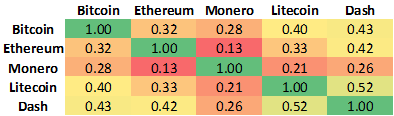Processed with 180 historical historical data after August 29, 2017

This indicates that the Markowitz model could add value and perform better for individual asset analysis, or even a portfolio weighed equally in terms of risk adjustment. However, before we get results, you need to see a simple analysis of the expected profits and risk characteristics for each asset and for the Sharpe maximum portfolio. The information is illustrated in the following table: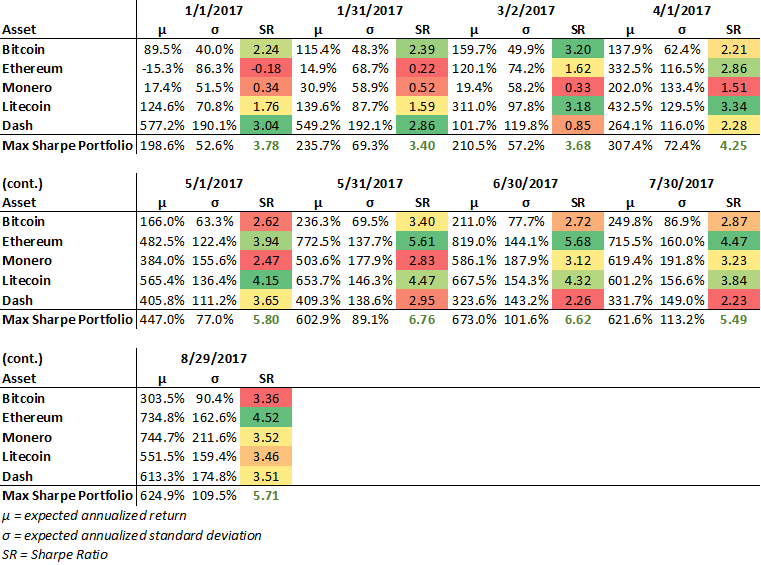Summary of assets data for each rebalancing period. Sharpe processed report assuming a 0% free risk rate for simplification

Using the characteristics of each individual asset and the correlation between them, you may process the profit and risk of any possible portfolio. However, in order to limit the results for viable portfolios, weights of positive weights were used and their total amount was equal to 100%. Then, Markowitz generated 50000 random portfolios and processed its profits and risk profile. Portfolios with maximum profits for each level of risk generated is known as the efficiency limit. On the edge of efficiency, we have a portfolio that has the highest profit per unit of risk. This is Sharpe’s maximum portfolio.

The profit and risk of the 50,000 random portfolios is shown in the graph below. Sharpe’s maximum portrait is marked with a blue spur.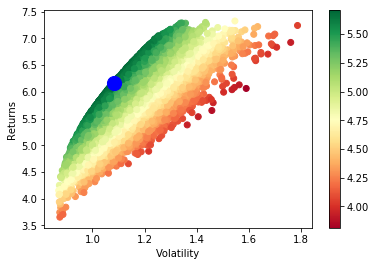Random portfolios and efficient border. Processed with 180 days of historical data from August 29, 2017.

The model is intended to add a larger proportion of the portfolio to assets with a higher individual Sharpe ratio, marked green in the table, and to avoid those with a relatively small or negative proportion, marked in red. Weights processed for the optimal portfolio are in line with expectations. These weights can be studied in the chart below: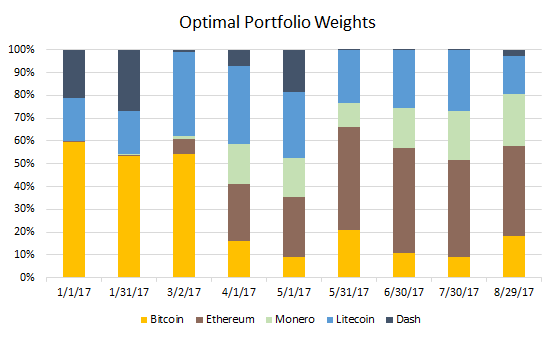Using these optimal weights, Markowitz made a Catalyst strategy test using all the available YTD data (from January 2017 in September 2017). He also tested an equally weighted portfolio portfolio and another test for a buying and retention strategy for each asset. Then, it processed the average annualized profits and the standard deviations for each strategy. The results are illustrated in the following table: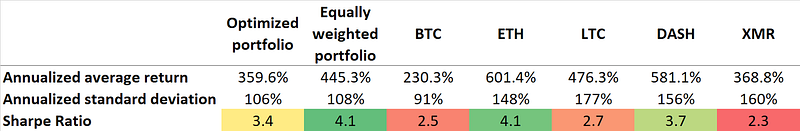### Summary of results

The optimized portfolio has a very good performance. It earned 359.6% annualized profits, with a standard deviation of 106%, which is a Sharpe report of 3.4. It is very high and would be difficult to obtain from investments in the major classes of assets (fixed income, equities, etc.). However, the optimized portfolio was not the best performance strategy. The best performance was the portfolio with equal weights.

Even more surprising, there were two buying and retention strategies for individual assets (ETH and DASH) that had better performance than the “optimal portfolio.” They do not comply with the MPT strategy and the benefits of diversification. One of the possible explanations is that the crypto market does not yet offer mature assets, so the correlation between them may not be quite stable to predict standard deviations of the portfolio.